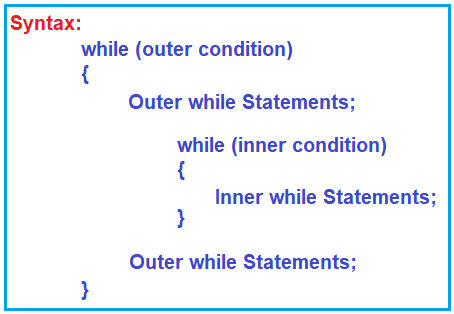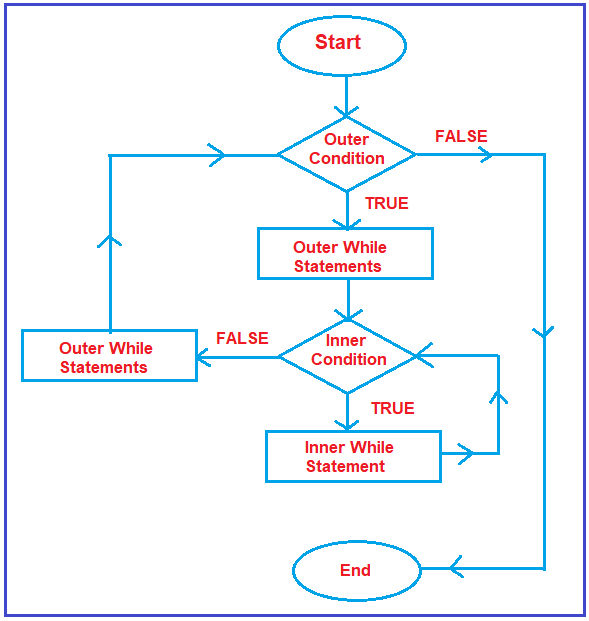# Nested While Loop in C

## Nested While Loop in C Programming Language with Examples

In this article, I am going to discuss Nested While Loop in C Programming Language with Definitions, Syntax, Flow Charts, and Examples. Please read our previous article, where we discussed While Loop in C Language with Examples.

##### Nested While Loop in C Programming Language:

Writing while loop inside another while loop is called nested while loop or you can say defining one while loop inside another while loop is called nested while loop. That is why nested loops are also called “loops inside the loop”. There can be any number of loops inside one another with any of the three combinations depending on the complexity of the given problem.

In implementation when we need to repeat the loop body itself n number of times then we need to go for nested loops. Nested loops can be designed for up to 255 blocks.

##### Nested While Loop Syntax in C Language:

Following is the syntax to use the nested while loop in C language.Note: In the nested while loop, the number of iterations will be equal to the number of iterations in the outer loop multiplied by the number of iterations in the inner loop which is almost the same as the nested for loop. Nested while loops are mostly used for making various pattern programs in C like number patterns or shape patterns.

##### Execution Flow of Nested While Loop in C Language:

The outer while loop executes based on the outer condition and the inner while loop executes based on the inner condition. Now let us understand how the nested while loop executes. First, it will check the outer loop condition and if the outer loop condition fails, then it will terminate the loop.

Suppose if the outer loop condition is true, then it will come inside, first, it will print the outer loop statements which are there before the inner loop. Then it will check the inner loop condition. If the inner while condition is true, then the control move inside and executes the inner while loop statements. After execution of inner while loop statements, again, it will check the inner while loop condition because it is a loop and as long as the condition is true, it will repeat this process. Once the inner while loop condition fails, then the control moves outside and executes the statements which are present after the inner while loop. Once it executes then, again it will go and check the outer while loop condition. And if it is true, then it will again execute the same process.

So, when the loop will terminate means when the outer while loop condition becomes false.

##### Flow Chart of Nested While Loop:

Please have a look at the following diagram, which represents the flow chart of the nested while loop.The flow will start and first, it will check the outer while loop condition. And if the outer while loop condition failed, then it will come to end. Suppose, the outer loop condition is true, then it will first execute the outer while loop statements if any. After execution of Outer while loop statements, it will check the inner while loop condition. For the inner while loop condition, it will also check for true and false. Suppose, the inner while loop condition is true, then inner while loop statements are executed. After executing the inner while loop statements, again, it will check the inner while loop condition, and this inner loop execution process will repeat as long as the inner while loop condition is true. If the inner while loop condition is false, then the remaining outer loop statements are executed. Once, the outer loop statements are executed, then again, it will come and check the outer while condition. This is the flow of the nested while loop.

##### Example: WAP to print the following format.###### Program:
```#include <stdio.h>
int main ()
{
int i, n, in;
printf ("ENTER  A NUMBER ");
scanf ("%d", &n);
i = 1;
while (i <= n)
{
printf ("\n");
in = 1;
while (in <= i)
{
printf ("%d ", in);
in = in + 1;
}
i = i + 1;
}
return 0;
}```
##### Example: WAP to print the following format:###### Program:
```#include <stdio.h>
int main()
{
int i, n, dn;
printf("ENTER  A NUMBER ");
scanf("%d", &n);
i = n;
while(i >= 1)
{
printf("\n");
dn = i;
while(dn >= 1)
{
printf("%d ", dn);
dn = dn - 1;
}
i = i - 1;
}
return 0;
}```
##### Example: WAP to print the following format:###### Program:
```#include <stdio.h>
int main ()
{
int a = 1, b = 1;
while (a <= 5)
{
b = 1;
while (b <= 5)
{
printf ("%d ", b);
b++;
}
printf ("\n");
a++;
}
return 0;
}```

1.2.